# Free Ncert Solution for Class 7 Maths Chapter 2 Fractions and Decimals Exercise 2.1

Fractions and Decimals, Class 7 Ex. 2.1;

New Ncert Solution for Class 7 Maths Chapter 2 Fractions and Decimals Free Solution;

Exercise 2.1

Which of the drawings (a) to (d) show.
(i)  2×(1/5)        (ii)  3×(2/3)          (iii)  2×(1/2)        (iv)  3×(1/4) ;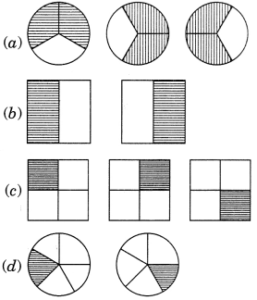Solution 1:-
(i) 2×(1/5) represents drawing (d);
(ii) 2×(1/2) represents drawing (b);
(iii) 3×(2/3) represents drawing (a);
(ic) 3×(1/4) represents drawing (c).

Question 2 :- Some pictures (a) to (c) are given below. Tell which of them show:

(i) 3 x (1/5) =  3/5;          (ii) 2 x (1/3) = 2/3;        (iii) 3 x (3/4) = 2(1/4);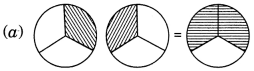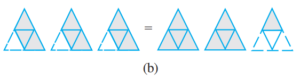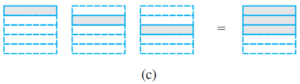Solution 2:-
(i) 3×(1/5) = 3/5 represents figure (c);
(ii) 2×(1/3) = 2/3 represents figure (a);
(iii) 3×(3/4)= 2(1/4) represents figure (b)

Question 3 :- Multiply and reduce to lowest form and convert into a mixed fraction: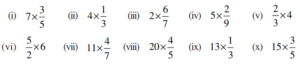Solution 3:-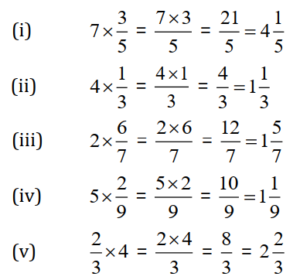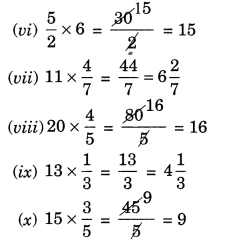(i) 1/2 of the circles in box (a)
(ii) 2/3 of the triangles in box (b)
(iii) 3/5 of the squares in box (c)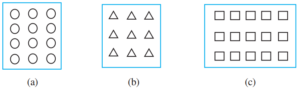Solution 4:-

(i) 1/2 of 12 circles = (1/2) x 12 = 6 circles;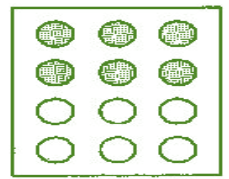(ii) 2/3 of triangles = (2/3) x 9 = 2 x 3 = 6 triangles;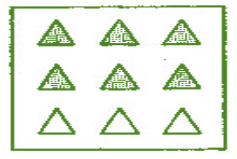(iii) 3/5 of squares = (3/5) x 15 = 3 x 3 = 9 squares;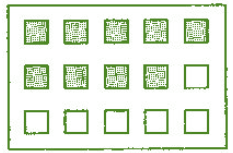Question 5 :- Find:

(a) 1/2 of   (i) 24 ;      (ii) 46 ;

(b) 2/3 of   (i) 18 ;      (ii) 27 ;

(c) 3/4 of   (i) 16 ;       (ii) 36 ;

(d) 4/5 of   (i) 20 ;       (iii) 35 ;

Solution 5:-

(a)  (i) 1/2 of 24 = (1/2) x 24 = 12 ;

(ii) 1/2 of 46 = (1/2) x 46 = 23 ;

(b) (i) 2/3 of 18 = (2/3) x 18 = 2 x 6 = 12 ;

(ii) 2/3 of 27 = (2/3) x 27 = 2 x 9 = 18;

(c) (i) 3/4 of 16 = (3/4) x 16 = 3 x 4 = 12;

(ii) 3/4 of 36 = (3/4) x 36 = 3 x 9 = 27;

(d) (i) 4/5 of 20 = (4/5) x 20 = 4 x 4 = 16;

(ii) 4/5 of 35 = (4/5) x 35 = 4 x 7 = 28;

Question 6 :- Multiply and express as a mixed fraction.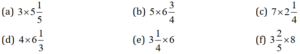Solution 6:-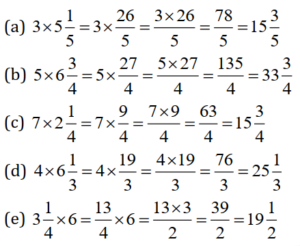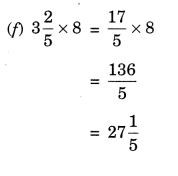Question 7 :- Find:

(a)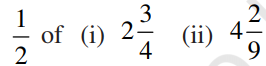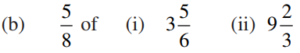Solution 7:-Question 8 :- Vidya and Pratap went for a picnic. Their mother gave them a water bottle that contained 5 litres of water. Vidya consumed 2/5 of the water. Pratap consumed the remaining water.
(i) How much water did Vidya drink?
(ii) What fraction of the total quantity of water did Pratap drink?

Solution 8:-

Total water in a bottle = 5 liters;

(i) Water consumed by Vidya = 2/5 of 5 litres
= (2/5) x 5 = 2 litres
water drink by Vidya = 2 litres.

(ii) Water consumed by Patap = 5 litres – 2 litres = 3 litres
Fraction of water consumed by Pratap = 1 – (2/5) = (5-2)/5 = 3/5.